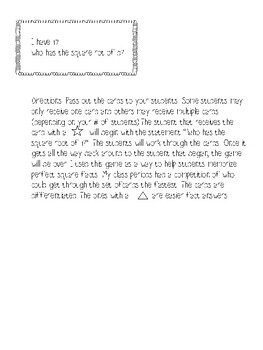# Perfect Square Roots - "I have, who has" gameSubject
Resource Type
Format
PDF (463 KB)
Standards
FREE
FREE

### Description

MGSE8.EE.2 Use square root and cube root symbols to represent solutions to equations. Recognize that x2 = p (where p is a positive rational number and lxl < 25) has 2 solutions and x3 = p (where p is a negative or positive rational number and lxl < 10) has one solution. Evaluate square roots of perfect squares < 625 and cube roots of perfect cubes > 1000 and < 1000.

"I have, who has?" game to help students memorize perfect square root fact

Total Pages
N/A
N/A
Teaching Duration
N/A
Report this Resource to TpT
Reported resources will be reviewed by our team. Report this resource to let us know if this resource violates TpT’s content guidelines.

### Standards

to see state-specific standards (only available in the US).
Use square root and cube root symbols to represent solutions to equations of the form 𝘹² = 𝘱 and 𝘹³ = 𝘱, where 𝘱 is a positive rational number. Evaluate square roots of small perfect squares and cube roots of small perfect cubes. Know that √2 is irrational.